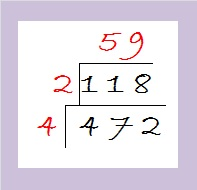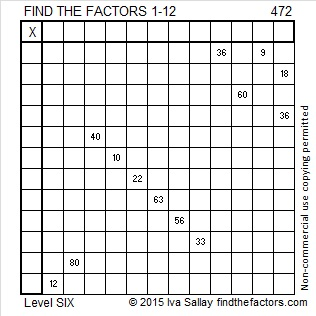# How to Simplify √472

472 is divisible by 4 because 72 can be evenly divided by 4, and that means that √472 can be simplified. This is the method I use to reduce it:

First I divide 472 by 4 and get 118. If I recognize that 118 has no square factors, I simply can say √472 = (√4)(√118) = 2√118.

If I don’t recognize that 118 has no other square factors, I can divide it by 2 to get 59 which is a prime number and definitely has no square factors besides the number 1.Then I take the square root of everything on the outside of the cake to get √472 = (√4)√(2 x 59) = (√4)(√118) = 2√118

Here is today’s puzzle:Print the puzzles or type the solution on this excel file:  12 Factors 2015-04-20

—————————————————————————————————

• 472 is a composite number.
• Prime factorization: 472 = 2 x 2 x 2 x 59, which can be written 472 = (2^3) x 59
• The exponents in the prime factorization are 3 and 1. Adding one to each and multiplying we get (3 + 1)(1 + 1) = 4 x 2 = 8. Therefore 472 has exactly 8 factors.
• Factors of 472: 1, 2, 4, 8, 59, 118, 236, 472
• Factor pairs: 472 = 1 x 472, 2 x 236, 4 x 118, or 8 x 59
• Taking the factor pair with the largest square number factor, we get √472 = (√4)(√118) = 2√118 ≈ 21.72556—————————————————————————————————